Updating search results...

# 18 Results

View
Selected filters:
• squareRating
0.0 stars

This three-part example from Illuminations highlights different aspects of students' understanding and use of patterns as they analyze relationships and make predictions, as discussed in the Algebra Standard. This first part, Making Patterns, includes an interactive figure for creating, comparing, and viewing multiple repetitions of patterns. The interactive figure illustrates how students can create pattern units of squares, then predict how patterns with different numbers of squares will appear when repeated in a grid, and check their predictions. In the second part, Describing Patterns, examples of various ways students might interpret the same sequence of cubes are given. This illustrates the importance of discussing and analyzing patterns in the classroom. The third part, Extending Pattern Understandings, demonstrates ways in which students begin to create a 'unit of units,' or a grouping that can be repeated, and begin to relate two patterns in a functional relationship. e-Math Investigations are selected e-examples from the electronic version of the Principles and Standards of School Mathematics (PSSM). The e-examples are part of the electronic version of the PSSM document. Given their interactive nature and focused discussion tied to the PSSM document, the e-examples are natural companions to the i-Math investigations.

Subject:
Mathematics
Material Type:
Activity/Lab
Interactive
Lesson Plan
Provider:
National Council of Teachers of Mathematics
Author:
National Council of Teachers of Mathematics
02/26/2019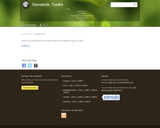Rating
0.0 stars

In this assessment task students are given a geoboard and rubberband and are asked to make a square, a triangle and a hexagon. Students can be assessed in a small group or whole class. A checklist and rubric are provided.

Subject:
Mathematics
Material Type:
Activity/Lab
Provider:
Hawaii State Department of Education
Author:
Hawaii State Department of Education
02/26/2019Conditional Remix & Share Permitted
CC BY-NC
Rating
0.0 stars
Rating
0.0 stars
Subject:
Mathematics
Material Type:
Full Course
Provider:
Pearson
11/02/2020Conditional Remix & Share Permitted
CC BY-NC
Rating
0.0 stars

Surface Area and Volume

Type of Unit: Conceptual

Prior Knowledge

Students should be able to:

Identify rectangles, parallelograms, trapezoids, and triangles and their bases and heights.
Identify cubes, rectangular prisms, and pyramids and their faces, edges, and vertices.
Understand that area of a 2-D figure is a measure of the figure's surface and that it is measured in square units.
Understand volume of a 3-D figure is a measure of the space the figure occupies and is measured in cubic units.

Lesson Flow

The unit begins with an exploratory lesson about the volumes of containers. Then in Lessons 2–5, students investigate areas of 2-D figures. To find the area of a parallelogram, students consider how it can be rearranged to form a rectangle. To find the area of a trapezoid, students think about how two copies of the trapezoid can be put together to form a parallelogram. To find the area of a triangle, students consider how two copies of the triangle can be put together to form a parallelogram. By sketching and analyzing several parallelograms, trapezoids, and triangles, students develop area formulas for these figures. Students then find areas of composite figures by decomposing them into familiar figures. In the last lesson on area, students estimate the area of an irregular figure by overlaying it with a grid. In Lesson 6, the focus shifts to 3-D figures. Students build rectangular prisms from unit cubes and develop a formula for finding the volume of any rectangular prism. In Lesson 7, students analyze and create nets for prisms. In Lesson 8, students compare a cube to a square pyramid with the same base and height as the cube. They consider the number of faces, edges, and vertices, as well as the surface area and volume. In Lesson 9, students use their knowledge of volume, area, and linear measurements to solve a packing problem.

Subject:
Geometry
Mathematics
Provider:
Pearson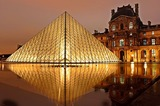Conditional Remix & Share Permitted
CC BY-NC
Rating
0.0 stars

Lesson OverviewStudents use scissors to transform a net for a unit cube into a net for a square pyramid. They then investigate how changing a figure from a cube to a square pyramid affects the number of faces, edges, and vertices and how it changes the surface area and volume.Key ConceptsA square pyramid is a 3-D figure with a square base and four triangular faces.In this lesson, the net for a cube is transformed into a net for a square pyramid. This requires cutting off one square completely and changing four others into isosceles triangles.It is easy to see that the surface area of the pyramid is less than the surface area of the cube, because part of the cube's surface is cut off to create the pyramid. Specifically, the surface area of the pyramid is 3 square units, and the surface area of the cube is 6 square units. Students will be able to see visually that the volume of the pyramid is less than that of the cube.Students consider the number of faces, vertices, and edges of the two figures. A face is a flat side of a figure. An edge is a segment where 2 faces meet. A vertex is the point where three or more faces meet. A cube has 6 faces, 8 vertices, and 12 edges. A square pyramid has 5 faces, 5 vertices, and 8 edges.Goals and Learning ObjectivesChange the net of a cube into the net of a pyramid.Find the surface area of the pyramid.

Subject:
Geometry
Material Type:
Lesson Plan
Author:
Pearson
11/02/2020Rating
0.0 stars

In this lesson plan, students are tasked with designing a playground at their school. Each section has its own requirement for each quadrilateral. In the end, they must be able to defend their shape against a classmate's of why they choose the shape. They must also list any other quadrilateral that could be drawn in that space.

Subject:
Mathematics
Material Type:
Lesson Plan
Provider:
Utah Education Network
02/26/2019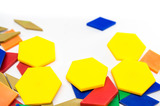Unrestricted Use
CC BY
Rating
0.0 stars

This resource is from Tools4NCTeachers.In this lesson, students explore attributes of polygons in order to create 2D shapes using given attributes.&nbsp; Students need some basic background knowledge of the attributes of polygons, including angles, sides, vertices, and right angles.&nbsp;&nbsp;Remix this lesson to include sample student work... Sharing is caring :)

Subject:
Mathematics
Material Type:
Activity/Lab
Lesson
Lesson Plan
Author:
DAWNE COKER
06/24/2020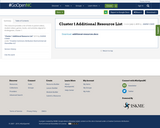Conditional Remix & Share Permitted
CC BY-NC-SA
Rating
0.0 stars

This resource is part of Tools4NCTeachers.

This list contains links to parent videos, student videos, games, books, and activities aligned to Kindergarten, Cluster 1.

Subject:
Mathematics
Material Type:
Activity/Lab
Game
11/06/2019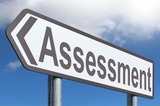Unrestricted Use
CC BY
Rating
0.0 stars

This resource is from Tools4NCTeachers.A Common Formative Assessment (CFA) provides a snapshot of current student understanding. It serves as a checkpoint, and may be used to guide future whole and small group instruction. This CFA addresses 2nd grade, Cluster 9&nbsp;standards. &nbsp;Answer key is provided.

Subject:
Mathematics
Material Type:
Assessment
Formative Assessment
Author:
DAWNE COKER
06/16/2020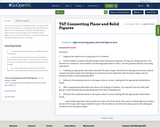Conditional Remix & Share Permitted
CC BY-NC-SA
Rating
0.0 stars

This resource is part of Tools4NCTeachers.

The goal of this lesson is for students to understand that plane figures are different from solid figures. Students will identify and locate the face of a solid figure that matches a plane figure, such as the face of a cube is a square, the base of a triangular based pyramid is a triangle.

Subject:
Mathematics
Material Type:
Lesson
Lesson Plan
11/06/2019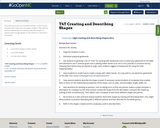Conditional Remix & Share Permitted
CC BY-NC-SA
Rating
0.0 stars

This resource is part of Tools4NCTeachers.

In this lesson, students will create and name given shapes. They will use precise mathematical vocabulary describe shapes to partner with enough details for the partner to recreate it accurately.

Subject:
Mathematics
Material Type:
Lesson
Lesson Plan
11/06/2019Unrestricted Use
CC BY
Rating
0.0 stars

This resource is from Tools4NCTeachers.&nbsp;&nbsp;This is a set of two&nbsp;tasks, which may be&nbsp;used for instruction or assessment.&nbsp; Each file contains a separate task,&nbsp;rubric, and recording sheets.&nbsp;&nbsp;Remix this resource to include extension ideas or addtional tasks.

Subject:
Mathematics
Material Type:
Activity/Lab
Assessment
Formative Assessment
Author:
DAWNE COKER
06/09/2020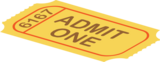Unrestricted Use
CC BY
Rating
0.0 stars

This resource is part of Tools4NCTeachers.This packet contains exit tickets for the First Grade stnanard G.3 (partitioning shapes). Exit tickets are written responses to questions posed at the end of a lesson. They are brief assessments which allow the teacher to determine student understanding of the concepts and skills taught that day.&nbsp;

Subject:
Mathematics
Material Type:
Assessment
Formative Assessment
Self Assessment
Author:
DAWNE COKER
06/14/2020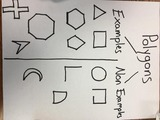Unrestricted Use
CC BY
Rating
0.0 stars

This resource is from Tools4NCTeachers. This file contains sample anchor charts to create with students. Anchor charts are to be made with students, then displayed as a classroom reference. Parents may also benefit from seeing anchor charts, as they provide a snapshot into the math class.Remix this resource to include pictures of your own anchor charts.

Subject:
Mathematics
Material Type:
Activity/Lab
Diagram/Illustration
Reference Material
Author:
DAWNE COKER
06/21/2020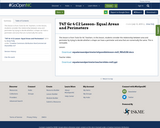Conditional Remix & Share Permitted
CC BY-NC-SA
Rating
0.0 stars

This lesson is from Tools for NC Teachers. In this lesson, students consider the relationship between area and perimeter by trying to decide whether a shape can have a perimeter and area that are numerically the same.

Subject:
Mathematics
Material Type:
Activity/Lab
Lesson Plan
11/06/2019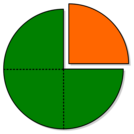Unrestricted Use
CC BY
Rating
0.0 stars

This resource is from Tools4NCTeachers.&nbsp;&nbsp;In this lesson students are introduced to the vocabulary associated with partitioning shapes into equal shares. Students will also explore shapes that are partitioned into halves, thirds, and fourths. Student recording sheets and pictures of anchor charts are included within this lesson.&nbsp;

Subject:
Mathematics
Material Type:
Activity/Lab
Lesson
Lesson Plan
Author:
DAWNE COKER
06/24/2020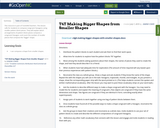Conditional Remix & Share Permitted
CC BY-NC-SA
Rating
0.0 stars

This resource is part of Tools4NCTeachers.

This lesson calls for students to compose congruent hexagons using smaller pattern block pieces. They will explain which arrangements of pattern block pieces compose a congruent hexagon, and count the number of smaller pieces that compose the "bigger" shape.

Subject:
Mathematics
Material Type:
Lesson
Lesson Plan
11/06/2019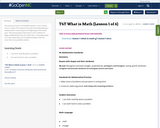Conditional Remix & Share Permitted
CC BY-NC-SA
Rating
0.0 stars

This resource is part of Tools4NCTeachers.

This is lesson one in a series of six lessons focused around developing a mathematical community at the beginning of the school year. The primary goal of this lesson is for students to begin establishing norms for math class. A secondary goal is to start exploring a non-standard problem that has more than one possible solution.

Subject:
Mathematics
Material Type:
Lesson
Lesson Plan